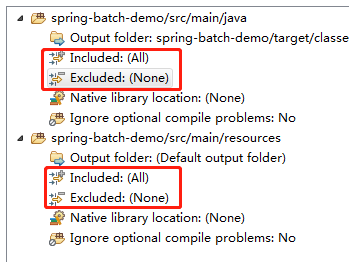eclipse无错误，java文件找不到或无法载入主要类，求大佬指点

package CheckCarWeight;

interface ComputerWeight {
public double computeWeight();
}
class Television implements ComputerWeight {
public double computeWeight() {return 3.5; //重写computeWeight()方法
}
class Computer implements ComputerWeight {
public double computeWeight() {return 2.67; //重写computeWeight()方法
}

class WashMachine implements ComputerWeight {
public double computeWeight() {return 13.8; //重写computeWeight()方法
}
class Truck {
ComputerWeight [] goods;
double totalWeights=0;
Truck(ComputerWeight[] goods) {
this.goods=goods;
}
public void setGoods(ComputerWeight[] goods) {
this.goods=goods;
}
public double getTotalWeights() {
totalWeights=0;
for (int i=0;i<goods.length;i++) {totalWeights+=goods[i].computeWeight();} //计算totalWeights
}

}
public class CheckCarWeight {
public void main(String args[]) {
ComputerWeight[] goods=new ComputerWeight; //650件货物
for(int i=0;i<goods.length;i++) { //简单分成三类
if(i%3==0)
goods[i]=new Television();
else if(i%3==1)
goods[i]=new Computer();
else if(i%3==2)
goods[i]=new WashMachine();
}
Truck truck=new Truck(goods);
System.out.printf("\n货车装载的货物重量:%-8.5f kg\n",truck.getTotalWeights());
goods=new ComputerWeight; //68件货物
for(int i=0;i<goods.length;i++) { //简单分成两类
if(i%2==0)
goods[i]=new Television();
else
goods[i]=new WashMachine();
}
truck.setGoods(goods);
System.out.printf("货车装载的货物重量:%-8.5f kg\n",truck.getTotalWeights());
}
}}}}

3个回答

public void main(String args[]) ，这个main方法得是static的，写成这样 public static void main(String args[])public static void main(String args[]) 程序执行的入口